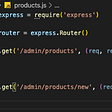# Numpy Operations

`np.insert` - To Insert items to an array

`np.append` - To append items to an array

`np.delete` - To delete items from an array

`np.unique` - To get unique items from an array

A np.insert(arr, obj, values, axis)

• arr: Input array
• obj : The index before which insertion is to be made
• values: The array of values to be inserted
• axis: The axis along insert in given array. If not given, arr is flattened
`>>>a = np.random.randint(10,20,(2,3,4)) # Axis 0–2 ; Axis 1–3 ; Axis 2–4 Now we will insert in different #indexes using np.insert operation>>>print(a)>>>print(a.shape)[[[13 13 18 11]  [19 11 18 16]  [15 10 13 18]] [[12 16 14 14]  [11 14 11 13]  [17 17 15 14]]] (2, 3, 4)#Insert along axis =0>>>np.insert(a,1,100,axis=0)array([[[ 13,  13,  18,  11],        [ 19,  11,  18,  16],        [ 15,  10,  13,  18]],       [[100, 100, 100, 100],        [100, 100, 100, 100],        [100, 100, 100, 100]],       [[ 12,  16,  14,  14],        [ 11,  14,  11,  13],        [ 17,  17,  15,  14]]])# Insert along axis = 1>>>np.insert(a,0,50,axis=1)array([[[50, 50, 50, 50],         [13, 13, 18, 11],         [19, 11, 18, 16],         [15, 10, 13, 18]],        [[50, 50, 50, 50],         [12, 16, 14, 14],         [11, 14, 11, 13],         [17, 17, 15, 14]]])# Insert along axis=2>>>np.insert(a,0,200,axis=2)array([[[200,  13,  13,  18,  11],        [200,  19,  11,  18,  16],        [200,  15,  10,  13,  18]],       [[200,  12,  16,  14,  14],        [200,  11,  14,  11,  13],        [200,  17,  17,  15,  14]]])`

B np.append(arr, values, axis)

• arr: Array
• values: To be appended to arr. It must be of the same shape as of arr (excluding axis of appending)
• axis: The axis along which append operation is to be done. If not given, both parameters are flattened
`#Example 1:>>>a = np.random.randint(2,6,(2,3,3))>>>print(a)>>>print(a.shape)[[[4 3 2] [3 5 3] [3 5 5]] [[2 4 3] [5 4 5] [5 2 3]]] (2, 3, 3)# Append a value to the array. It flattens the array and append -99 to the end>>>np.append(a, 50)array([ 4, 3, 2, 3, 5, 3, 3, 5, 5, 2, 4, 3, 5, 4, 5, 5, 2, 3, 50])#Example  2:>>>b = np.random.randint(2,6,(2,1,3))>>>print(b)[[[4 5 5]] [[3 5 3]]]>>>np.append(a,b,axis = 1)array([[[4, 3, 2], [3, 5, 3], [3, 5, 5], [4, 5, 5]],  [[2, 4, 3], [5, 4, 5], [5, 2, 3], [3, 5, 3]]])`

C np.delete(arr, obj, axis)

• arr: Input array
• obj: Can be a slice, an integer or array of integers, indicating the subarray to be deleted from the input array
• axis: The axis along which to delete the given subarray. If not given, arr is flattened
• Returns a copy of arr with the elements specified by obj removed. Note that delete does not occur in-place. If axis is None, out is a flattened array.
`>>>a = np.random.randint(2,6,(2,3,3))>>>print(a)>>>print(a.shape)[[[2 3 3] [2 3 4] [4 5 4]] [[3 2 4] [5 4 4] [5 4 3]]] (2, 3, 3)# Delete 0th element along Axis=0>>>np.delete(a,(0),axis = 0)array([[[3, 2, 4], [5, 4, 4], [5, 4, 3]]])# Delete 0th and 2nd element along axis=1>>>np.delete(a,(0,2),axis = 1)array([[[2, 3, 4]],        [[5, 4, 4]]])# Delete 0th and 1st element along axis=2>>>np.delete(a,(0,1),axis = 2)array([[,         ,         ],        [,         ,         ]])`

D np.unique(arr, return_index, return_inverse, return_counts)

• arr: Input array
• return_index : If True, returns the indices of elements in the input array.
• return_counts: If True, returns the number of times the element in unique array appears in the original array.
`#Initializing array>>>a = np.random.randint(2,20,(2,3,3))>>>print(a)>>>print(a.shape)[[[ 6 2 7] [ 7 9 15] [ 2 7 7]]  [[ 3 18 15] [ 4 7 9] [ 8 2 19]]] (2, 3, 3)>>>np.unique(a)array([ 2, 3, 4, 6, 7, 8, 9, 15, 18, 19])# return_counts= True>>>np.unique(a,return_counts=True) # Returns tuple(array([ 2, 3, 4, 6, 7, 8, 9, 15, 18, 19]), array([3, 1, 1, 1, 5, 1, 2, 2, 1, 1]))# return_index = True#1.unique values. 2. Their indexes. 3. Their counts.>>>np.unique(a,return_counts=True,return_index=True)(array([ 2, 3, 4, 6, 7, 8, 9, 15, 18, 19]), array([ 1, 9, 12, 0, 2, 15, 4, 5, 10, 17]), array([3, 1, 1, 1, 5, 1, 2, 2, 1, 1]))`

## Basic Operations & Functions

• `np.add()` - Adding two arrays

Arguments are the two arrays to be added.

• `np.subtract()` - Subtracting two arrays

Arguments are the two arrays to be added.

• `np.multiply()` - Multiplying two arrays

Arguments are the two arrays to be added.

• `np.divide()` - Dividing two arrays

Arguments are the two arrays to be added.

• `np.sum()` - Sum of items in an array

Argument is the array of which elements are to be added.

• `np.min()` - Minimum of items in an array

Argumet is the array from which max value is to be returned.

• `np.max()` - Minimum of items in an array

Argumet is the array from which max value is to be returned.

• `np.mean()` - Mean of items in an array

Argumet is the array from which mean value is to be returned.

• `np.sort()` - Sorting an array

Argument is the array which is to be sorted.

--

--

--

## More from omkar katare

Love podcasts or audiobooks? Learn on the go with our new app.

## How to Build a Simple and Interactive Bar Chart With React.js and CSS-in-JS## Search with Algolia in React Native using multiple instance search component## How to add Sentry with source maps to a React app## Web Socket: Gateway for developing real-time Apps## Angular Utilities: init Directive## P5 clock practice — Candle Boy## Python Object Oriented Programming Overview## USING DATETIME IN PYTHON